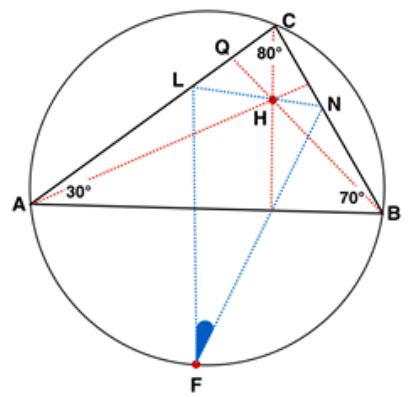# Find the angle $\angle NFL$

Geometry Level 4Let $\triangle ABC$ be an acute triangle with angles 30, 70 and 80 (degrees) at vertices $A$, $B$, $C$ respectively. Altitudes $AP, BQ$ and $CR$ intersects in the orthocenter $H$ of $\triangle ABC$. Points $N, L$ on sides $BC$ and $AC$ are such that segment $NL$ contains $H$ and $NL$ bisects $\angle AHQ$. The point $F$ on the circumcircle of $\triangle ABC$ bisects the smaller arc $AB$. Find $\angle NFL$ in degrees.

×